# Suppose T: ℝ3→ℝ2 is a linear transformation. Let U and V be the vectors given below, and suppose that T(U) and T(V) are...

Suppose T: ℝ3→ℝ2 is a linear transformation. Let U and V be the vectors given below, and suppose that T(U) and T(V) are as given. Find T(3U+3V).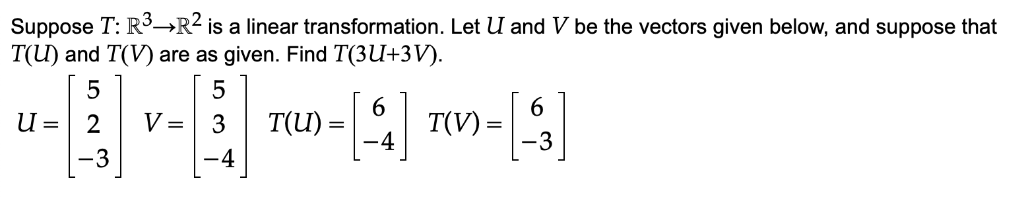Suppose T: R->R2 is a linear transformation. Let U and V be the vectors given below, and suppose that T(U) and T(V) are as given. Find T(3U+3V). 5 5 6 T(V) 6 =n 2 -3 T(U) V = 3 -4 3 -4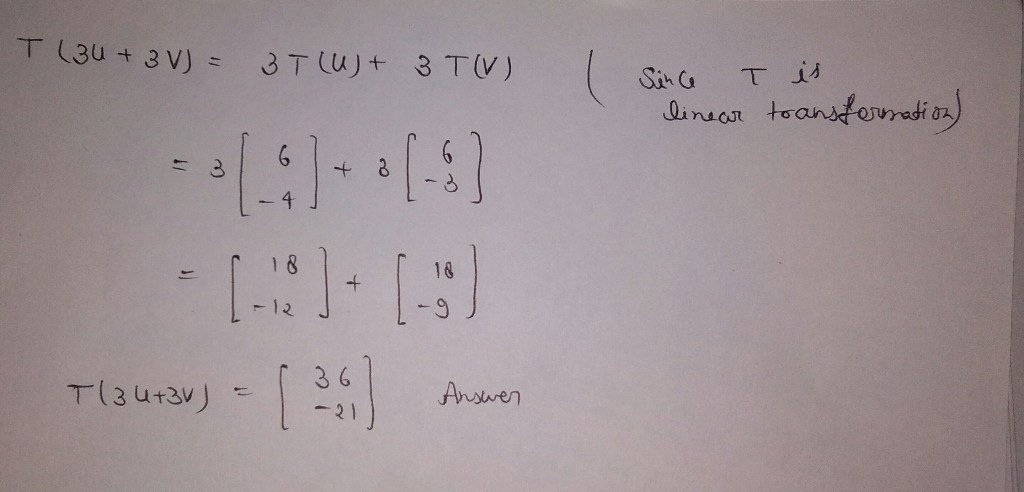##### Add Answer of: Suppose T: ℝ3→ℝ2 is a linear transformation. Let U and V be the vectors given below, and suppose that T(U) and T(V) are...
Similar Homework Help Questions
• ### Problem 1. The figure below shows the vectors u, v, and w, along with the images...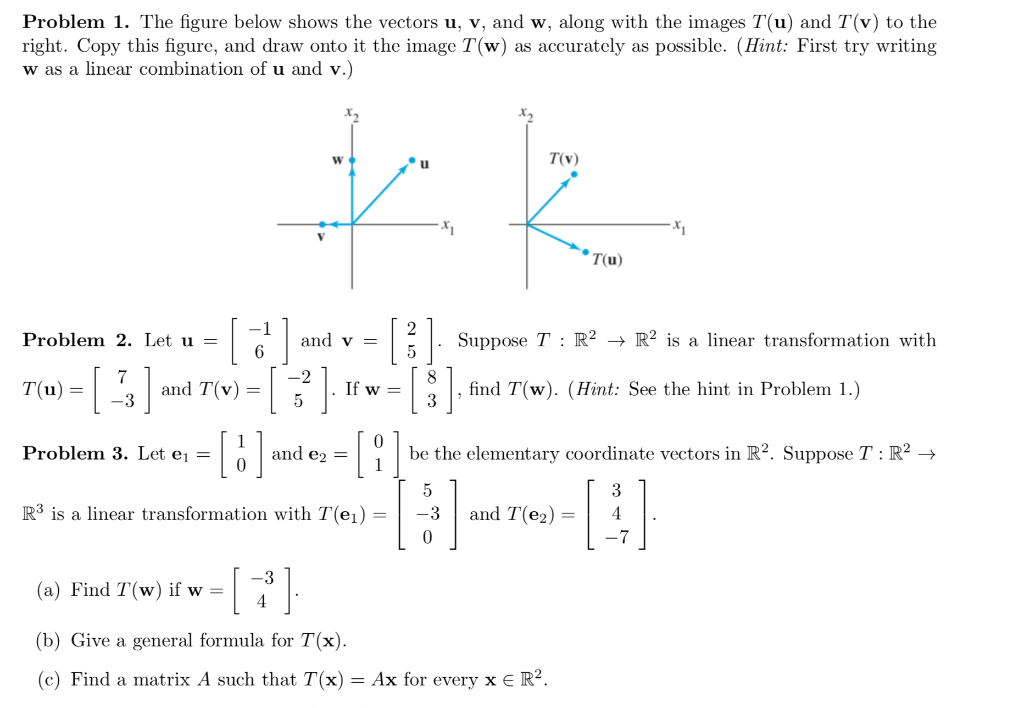Problem 1. The figure below shows the vectors u, v, and w, along with the images T(u) and T(v) to the right. Copy this figure, and draw onto it the image T(w) as accurately as possible. (Hint: First try writing w as a linear combination of u and v.) TV (u) Problem 2. Let u = | and v Suppose T : R2 + R2 is a linear transformation with 6 1 3) Tu = T(u) = -3 and T(v)...

• ### Let u and v be the vectors shown in the figure to the right, and suppose...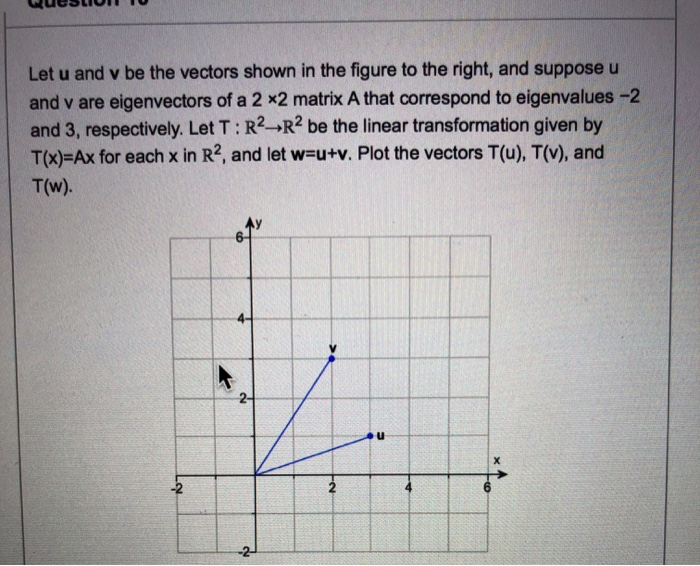Let u and v be the vectors shown in the figure to the right, and suppose u and v are eigenvectors of a 2 x2 matrix A that correspond to eigenvalues -2 and 3, respectively. Let T: R2 R2 be the linear transformation given by T(x)-Ax for each x in R2, and let w-u+v. Plot the vectors T(u), T(v), and T(w). 2- u -2 2 4 -2 10- T(v) T(w -10 10 T(u) -10- Ay 10- T(v) T(w) T(u) 10...

• ### Problem 4. Let V be a vector space and let T : V → V and U : V → V be two linear transforinations...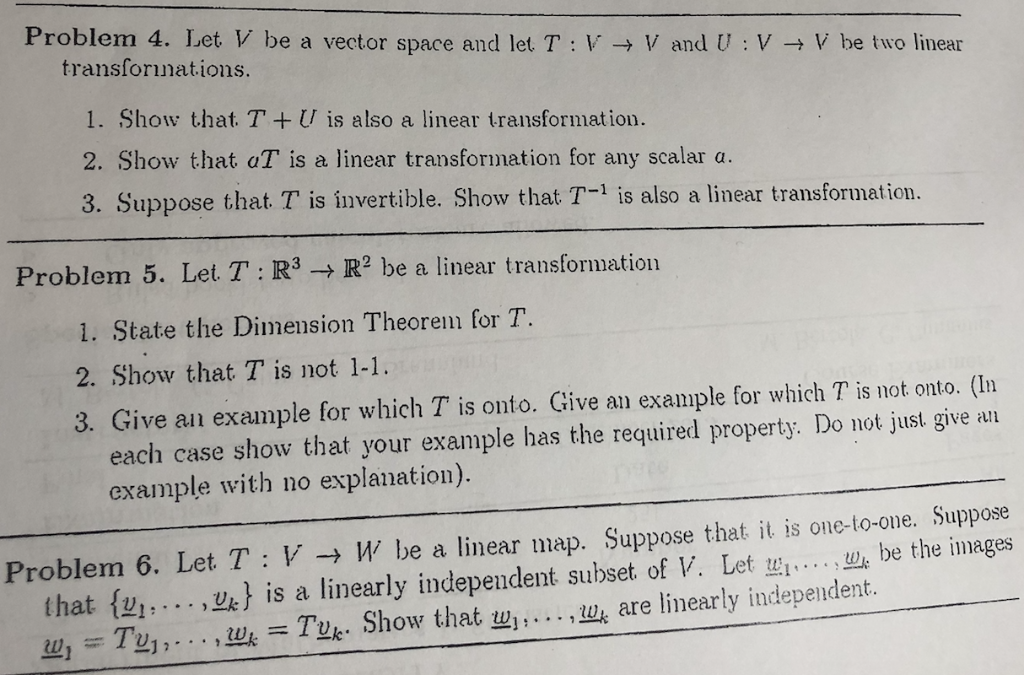I need the answer to problem 6 Clear and step by step please Problem 4. Let V be a vector space and let T : V → V and U : V → V be two linear transforinations 1. Show that. TU is also a linear transformation. 2. Show that aT is a linear transformation for any scalar a. 3. Suppose that T is invertible. Show that T-1 is also a linear transformation. Problem 5. Let T : R3 →...

• ### For each transformation below, find the value of T(U). 1) Let T be a linear transformation...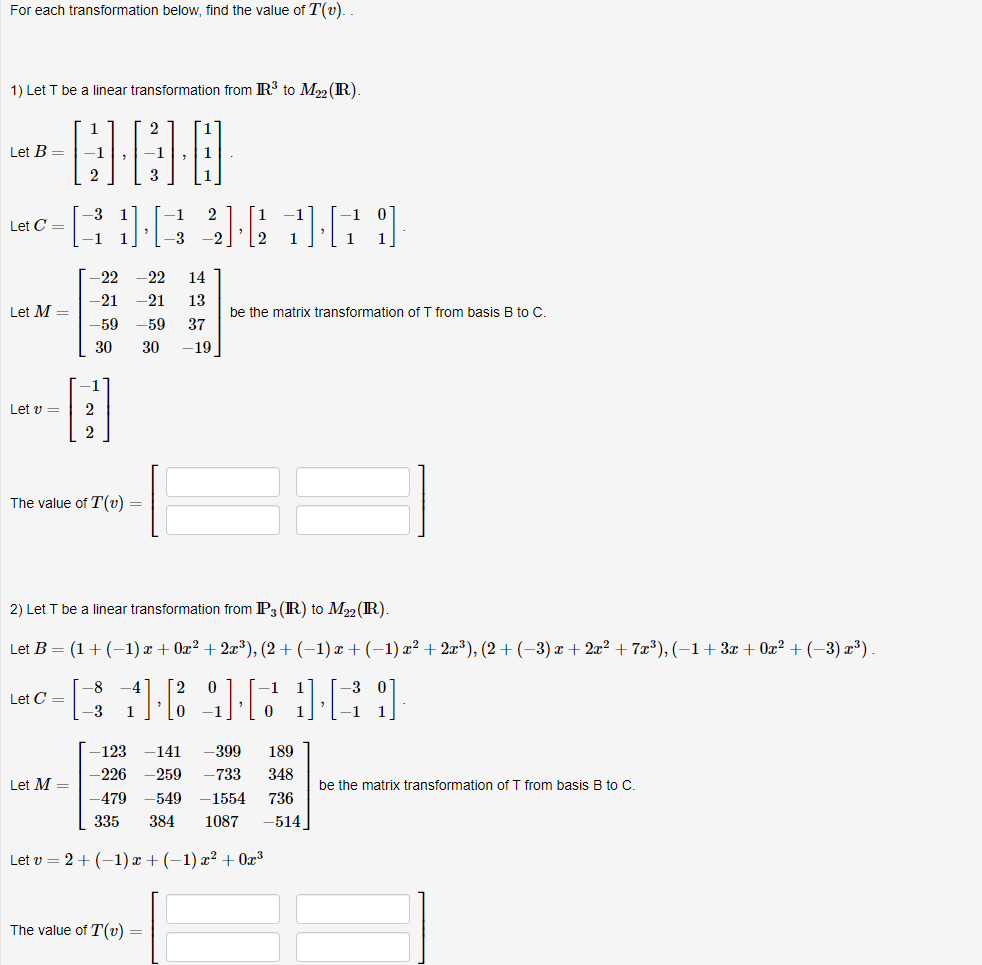For each transformation below, find the value of T(U). 1) Let T be a linear transformation from R\$ to M2 (R) 2 Let B= -1 2 3 Let C= .  1 -22 -21 -22 -21 -59 14 13 Let M= be the matrix transformation of T from basis B to C 37 -59 30 30 -19 -1 Let v= 2 2 The value of T(0) = 2) Let T be a linear transformation from P3 (R) to M22(R). Let...

• ### Find 2u, -3v, u + v, and 3u - 4v for the given vectors u and...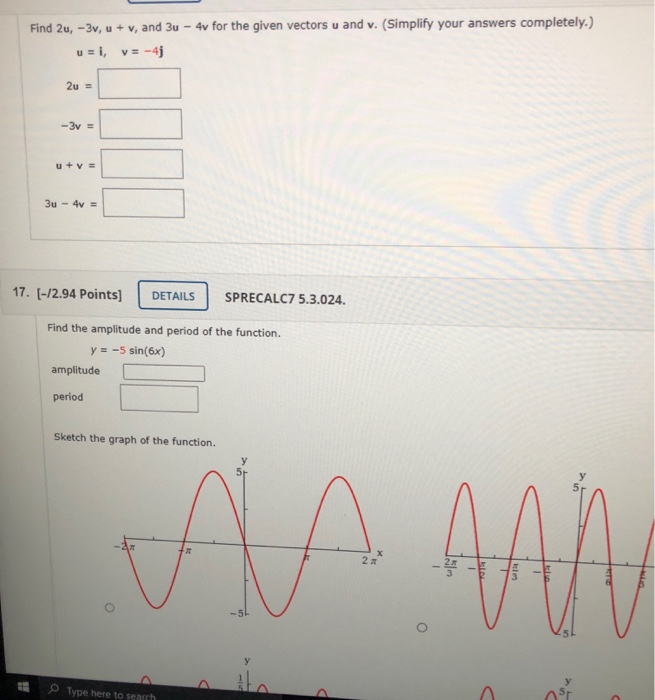Find 2u, -3v, u + v, and 3u - 4v for the given vectors u and v. (Simplify your answers completely.) u = i, v= -4j 2u = -3v = u + V = 3u - 4 = 17. [-12.94 Points) DETAILS SPRECALC75.3.024. Find the amplitude and period of the function. y = -5 sin(6x) amplitude period Sketch the graph of the function. AA Am Type here to search A

• ### 7. Let T : V → W be a linear transformation, and let v1,v2,...,vn be vectors...

7. Let T : V → W be a linear transformation, and let v1,v2,...,vn be vectors in V. Suppose that T (v1), T (v2), . . . , T (vn) are linearly independent. Show that v1, v2, . . . , vn are linearly independent.

• ### Please give answer with the details. Thanks a lot! Let T: V-W be a linear transformation between vector spaces V and W...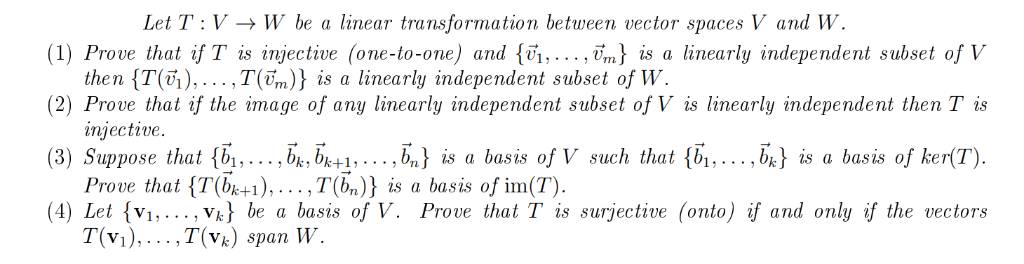Please give answer with the details. Thanks a lot! Let T: V-W be a linear transformation between vector spaces V and W (1) Prove that if T is injective (one-to-one) and {vi,.. ., vm) is a linearly independent subset of V the n {T(6),…,T(ền)} is a linearly independent subset of W (2) Prove that if the image of any linearly independent subset of V is linearly independent then Tis injective. (3) Suppose that {b1,... bkbk+1,. . . ,b,) is a...

• ### For each transformation below, find the closed form of the transformation. 1) Let T be a...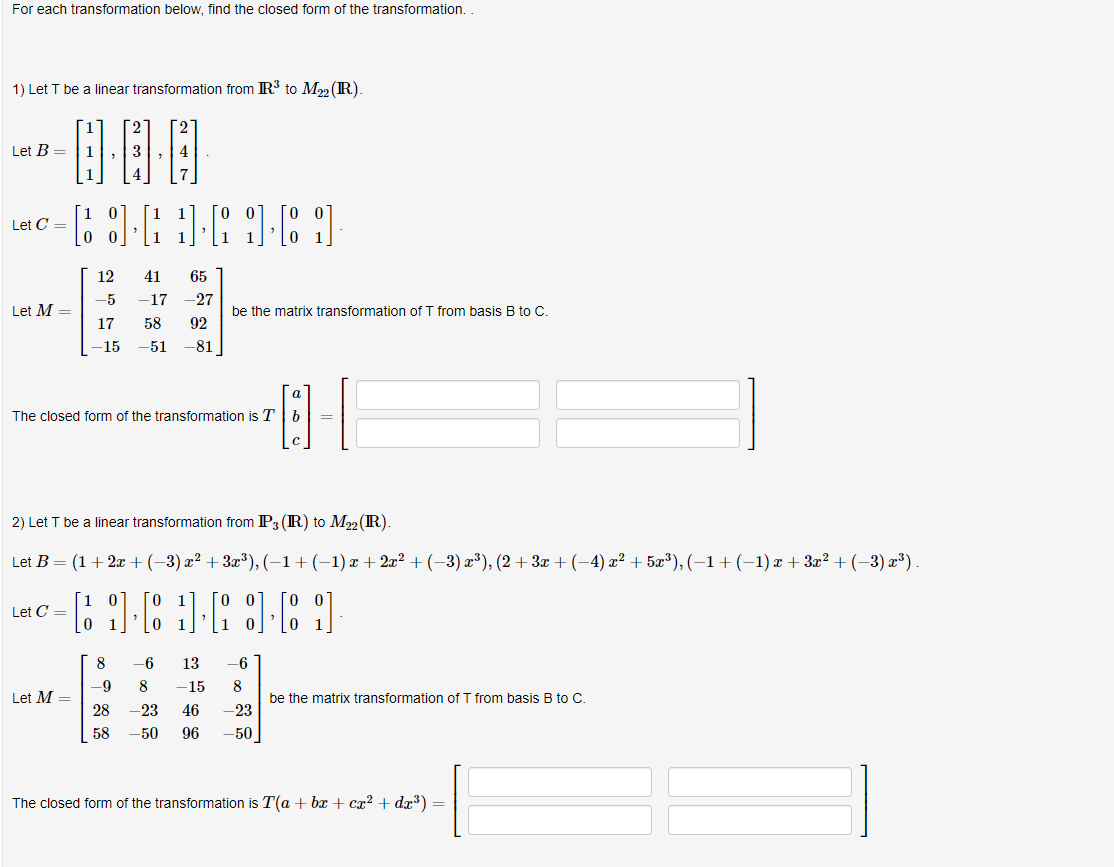For each transformation below, find the closed form of the transformation. 1) Let T be a linear transformation from R\$ to M22 (R) [i Let B=1 0:00 [. :]   [0 ] Let C= 12 41 -17 -5 65 -27 92 Let M = be the matrix transformation of T from basis B to C 17 58 -15 -51 81 The closed form of the transformation is Tb 3-1 2) Let T be a linear transformation from P3(R) to...

• ### 1- Two vectors are given as u = 2 – 5j and v=-{+3j. a- Find the...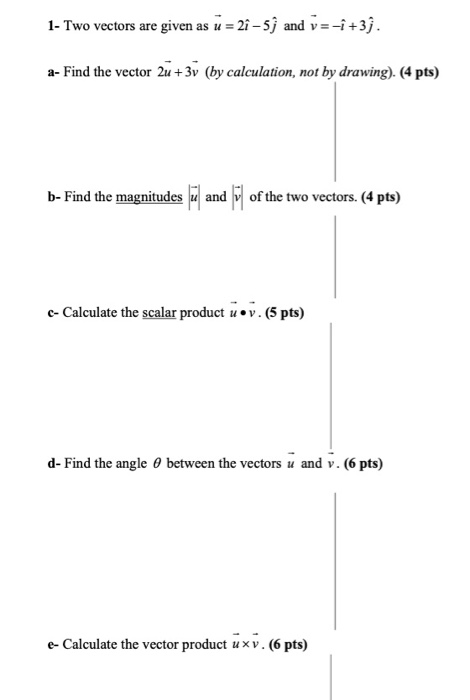1- Two vectors are given as u = 2 – 5j and v=-{+3j. a- Find the vector 2u +3v (by calculation, not by drawing). (4 pts) b- Find the magnitudes luand il of the two vectors. (4 pts) c- Calculate the scalar product u•v. (5 pts) d- Find the angle between the vectors u and v. (6 pts) - Calculate the vector product uxv. (6 pts)

• ### Let T: R3 → R3 be the linear transformation that projects u onto v = (9,...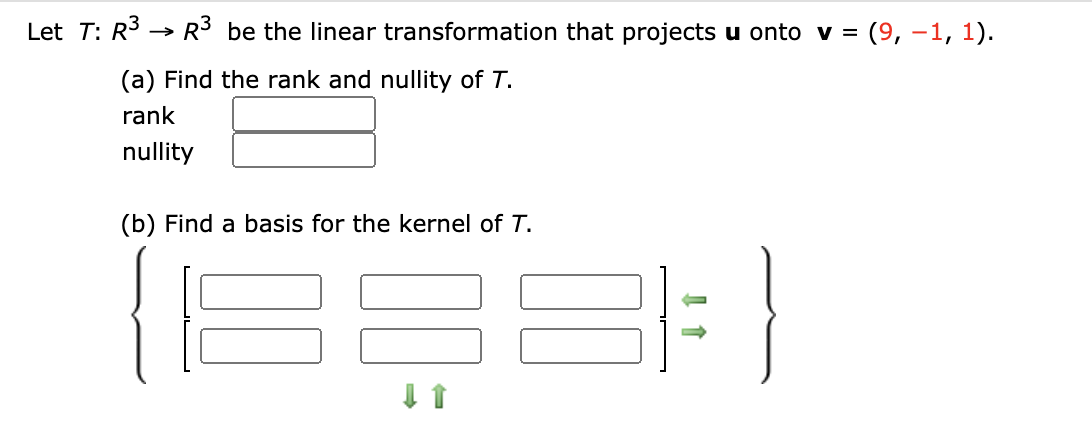Let T: R3 → R3 be the linear transformation that projects u onto v = (9, -1, 1). (a) Find the rank and nullity of T. rank nullity (b) Find a basis for the kernel of T.

Free Homework App